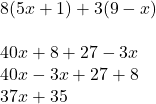## Which algebraic expression is equivalent to the expression below? 8(5x + 1) + 3(9 – x) A. 37x – 35 B. 37x + 19 C. 37x + 35 D. 43x + 35

Question

Which algebraic expression is equivalent to the expression below? 8(5x + 1) + 3(9 – x) A. 37x – 35 B. 37x + 19 C. 37x + 35 D. 43x + 35

in progress 0
1 month 2021-07-31T09:06:37+00:00 1 Answers 2 views 0